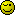# Simple (?) JOIN question

I have a question how to have two JOINs using the same table.
The previous sentence probably doesn’t make sense, so let me try to explain using an example. Let say, I have two tables

``````
CREATE TABLE users (
userid INTEGER AUTO_INCREMENT,
name VARCHAR(36),
PRIMARY KEY (userid)
)

CREATE TABLE transactions (
transactionid INTEGER AUTO_INCREMENT,
sellerid INTEGER,
price INTEGER,
PRIMARY KEY (transactionid)
)

``````

Now, when I want buyer information for all transactions where the price was greater than 10, I could retrieve this using the following statement

``````
SELECT
FROM
WHERE
transactions.price > 10

``````

But what statement should I use when I also want to retrieve the sellers name with the same query?

``````
seller.name as seller
join  transactions
on transaction.sellerid = seller.userid
where transactions.price > 10

``````

i prefer to write the tables in the FROM clause in a logical sequence

``````SELECT buyer.name AS buyer
, seller.name AS seller
FROM transactions
INNER
INNER
ON seller.userid = transactions.sellerid
WHERE transactions.price > 10
``````

i always try to start the FROM clause with the “driving” table, i.e. the one mentioned in the WHERE clause which has the main restriction, the one which the other tables are joined to

great, your responses make it all clear.
I missed the point that I should give the table that is being joined an alias, so that I can join it twice

I actually would like to ask a second question and that is about the efficiency of JOIN statements.

Let say, the transaction table has many records. And I would like to get the buyer and seller name for a specific transaction (eg transaction 123456). As I have been explained, I can do this in one query:

``````
SELECT
sellers.name AS seller
FROM
transactions
ON transactions.sellerid = sellers.userid
WHERE
transactions.transactionid = 123456

``````

Now, I would “visualize” this query as a large table being constructed (with buyer and seller names for all transactions) to which the WHERE clause is being applied.

If the database would indeed process the query like that, it would be very inefficient. In that case it would be better to obtain the buyerid and sellerid, and get the names in separate query.

Does anyone know if one should take these kind of considerations into account? Or can you expect the database to optimize the query?

yes, i dohave you ever done an EXPLAIN? which database system is this?

You take these considerations into account? Or you know the answer?No I have never done an EXPLAIN… I am not running a large database, it is more an academic interest. Hence, the stylized example.

I am using MySQL.

i know the answerthe reason i asked which database you’re using is because we have a mysql forum (for mysql, eh) and a databases forum (for everything else), and you didn’t post in the mysql forum and you also didn’t say which database you’re using

in your query, put the word EXPLAIN in front of the word SELECT, run it, and show us the results

Ok, so I have created the following php script to populate the tables with some random data

``````
\$seed = 100;
srand(\$seed);

// Fill users table with 1000 users
for (\$i = 0; \$i < 1000; \$i++) {
\$name = rand(0, 100000);
\$sql = "INSERT INTO users (name) VALUES ('\$name')";
mysql_query(\$sql, \$con);
}

// Fill transactions table with 200k transactions
for (\$i = 0; \$i < 200000; \$i++) {
while ((\$sellerid = rand(0, 999)) == \$buyerid);
\$price = rand(1, 100);
\$sql = "INSERT INTO transactions (buyerid, sellerid, price) ";
\$sql .= "VALUES (\$buyerid, \$sellerid, \$price)";
mysql_query(\$sql, \$con);
}

``````

Now executing the SELECT statement with two JOINs returns buyer=72396 (corresponding to userid=148) and seller=88733 (corresponding to userid=184) pretty instantaneously.

And EXPLAIN gives an output as per screenshot.

Based on the speed of returning the result I would think MySQL is optimizing.

And, then I would assume any other respectable database system would do the same.

yep, they dook great, then we can keep it general, and I might be posting in the right forum after allAny insights in what EXPLAIN can tell me?

it can tell you when certain operations are inefficient, e.g. table scans where the “key” column is empty

but it doesn’t tell me that the WHERE clause is executed before the JOINs (as seems to be the case)?

yes, the WHERE clause is applied in step 1 to the transaction table (using its PRIMARY key)

the “rows” column indicates that 1 row has been retrieved (instead of all of them)

after that, the other tables are joined